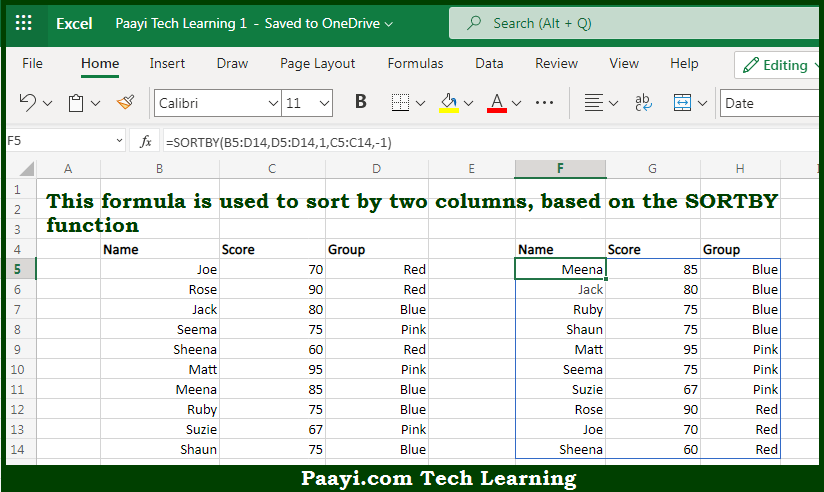# Learn How to Sort by Two Columns in Microsoft Excel

Written by | 0 Comments | 624 Views

In this article, you will learn how to evaluate things in Dynamic Arrays with formulas in Microsoft Excel using a single/combination(s) of functions. You will also know How to Sort by Two Columns and see the generic formula.

Learn How to Sort by Two Columns in Microsoft Excel

The main purpose of this formula is to sort by two columns. Here we will learn how to sort by two columns in the given data range in the workbook in Microsoft Excel. That implies, with the help of a formula based on the SORTBY function you can able to sort by two columns. So, with the help of this formula, you can able to sort by two columns in the given data range in the workbook in Microsoft Excel.

General Formula to Sort by Two Columns

=SORTBY(data,col1,order,col2,order)

The Explanation to Sort by Two ColumnsSo we know that with the help of the given formula above you can able to sort by two columns. Here we will learn how to sort by two columns in the given data range in the workbook in Microsoft Excel. As we know that the SORTBY function allows the sorting based on one or more "sort by" arrays, as long as dimensions are compatible with the source data. In the example given here, data is sorted in ascending order (A-Z) by default. This behavior is controlled by the sort_order arguments. So, with the help of this formula, you can be able to by two columns. So now you have learned how to sort by two columns, in the given data range in the workbook in Microsoft Excel.